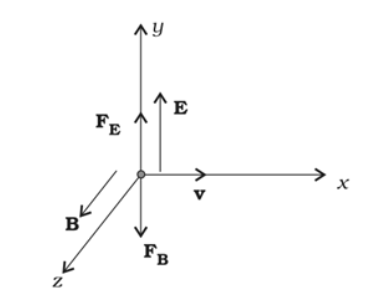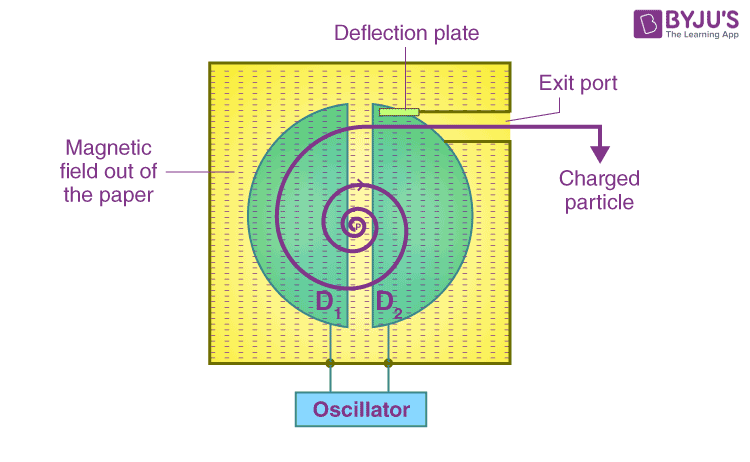Checkout JEE MAINS 2022 Question Paper Analysis : Checkout JEE MAINS 2022 Question Paper Analysis :

# Motion in Combined Electric And Magnetic Fields

An electric field is created by the electrical charge particle and is perpendicular to the magnetic field. The unit of the electric field is Volt/meter and is a vector quantity. We also know that the electric field is conservative in nature.

The magnetic field is the space around the magnet, where the magnetic force is observed. This field is created by the movement of the electric charges. Magnetic lines represent the direction of the magnetic field. The electric fields are generated around the particles, which are characterised by electric charges. The force created by the electric field is much stronger than the force created by the magnetic field. The orbiting motion of charges in a magnetic field is the basis for measuring the mass of an atom. A closed loop is formed by the magnetic field lines, and the electric field lines do not form a loop.

## Lorentz Force

• When a moving charge comes under the influence of magnetic and electric fields, it experiences Lorentz force. Lorentz force is a kind of force that can be calculated as the vector sum of forces created by magnetic and electric fields.
• Fnet = FE + FB
• Fnet = q(E + v x B)
• The below figure shows the representation of the electric field and the magnetic field along with the motion of charge when they are perpendicular to each other.• When the values of E and B are adjusted such that the magnitude of the two forces are equal, the total force acting on the charge is zero, and the charges will move in the field undeflected.
• When the strength of electric and magnetic fields are varied to get the forces due to electric and magnetic fields to be equal (FE = FB), then the charge can move in the field without any deflection.
• Fnet = 0
• FE = FB
• qE=Bqv
• E=Bv
• v=E/B
• This case is used when charged particles of a certain velocity (E/B) are used to pass through the crossed fields undeflected. This phenomenon is called a velocity selector. It was applied by J. Thomson to evaluate the charge to the mass ratio in 1897.
• Velocity selector is used in mass spectrometers, where charged objects are distinguished as per their charge to mass ratio.

## Cyclotron

A cyclotron is a machine used to accelerate charged particles or ions to high energies.

To enhance the energies of charged particles, cyclotron uses magnetic as well as electric fields. It is called crossed fields since the magnetic and electric fields are perpendicular to each other.The image above represents the cyclotron. A cyclotron consists of two flat and hollow semicircular metal disc-like containers represented as D1 and D2. These containers are separated by a narrow gap. Disc-like containers connected to a high-frequency oscillator have the capacity to generate an alternating voltage. Alternating electric field is created between D1 and D2 as well from D2 to D1. The charged particle in the containers is accelerated when it passes through the gap between the metal containers.

When a charged particle moves into a hollow semicircular metal disc-like container, it moves in a circular path and with a constant speed. Due to the shielding effect, the electric field is zero inside the container. The frequency of the cyclotron is independent of the speed of the charged particle and the radius of the circular path. These machines are used to bombard nuclei and are used to study nuclear reactions.

Stay tuned with BYJU’S for more such interesting derivations in physics, chemistry and maths in an engaging way with video explanations.

## Frequently Asked Questions – FAQs

### 1. What is a Cyclotron?

Cyclotron is a machine that is used to accelerate the charged ions or particles to high energies.

### 2. Strength of the electric field is proportional to?

Strength of the electric field is proportional to the electric charge.

### 3. What do the Van Allen radiation belts in space around the earth consist of?

They consist of energetic charges trapped in the magnetic field of the earth.

### 4. Which fields are used by cyclotrons?

Cyclotrons use both electric and magnetic fields.

### 5. What is a mass spectrometer?

Mass spectrometer is a device that finds its application in separating the charged particles based on their charge to mass ratio.